12. ATOMS

Q.1. State Bohr’s frequency condition.

Ans ⇒ It states that the frequency v of a radiation emitted on jumping of an electron from the outer orbit to the inner orbit of an atom is given by
E = hv = E2 – E1
Where E1 = total energy of the electron in the inner orbit and E2 = total energy of the electron in the outer orbit.

Q.2. Define distance of closest approach.

Ans ⇒ It is defined as the minimum distance from the centre of nucleus upto which an energetic ∝- particle travelling directly towards the nucleus can move during head-on-collision before coming to rest and retracing its path. It is denoted by r0.

Q.3. Define impact parameter.

Ans ⇒ It is defined as the perpendicular distance of the velocity vector of the a-particle from the centre if the nucleus when it is far away from the atom. It is denoted by b.

Q.4. Define energy level.

Ans ⇒ It is defined as the horizontal line which represents the total energy of an electron in a stationary orbit of an atom.

Q.5. How an energy level is represented ?

Ans ⇒ An energy level is represented by a horizontal line drawn according to some suitable energy scale.

Q.6. What are the limitations of Bohr’s atomic model ?

Ans ⇒ Limitations of Bohr’s atomic model are as follow :

(i) It could successfully explain the spectra of simple atoms having one electron but it could not explain the spectra of complex atoms having more than one electron.

(ii) It does not give any idea about the distribution of electrons in an atom.

(iii) This model could not account for the wave nature of electrons.

(iv) It does not give any idea about the relative intensity of spectral lines.

(v) It could not explain the splitting of spectral lines into a number of spectral lines under the effect of magnetic field.

Q.7. Draw a labelled diagram of experimental set up of Rutherford’s alpha particle scattering experiment. Write two important inferences drawn from this experiment.

Ans ⇒ The experimental set up of Rutherford’s a particle scattering experiment is shown in Fig.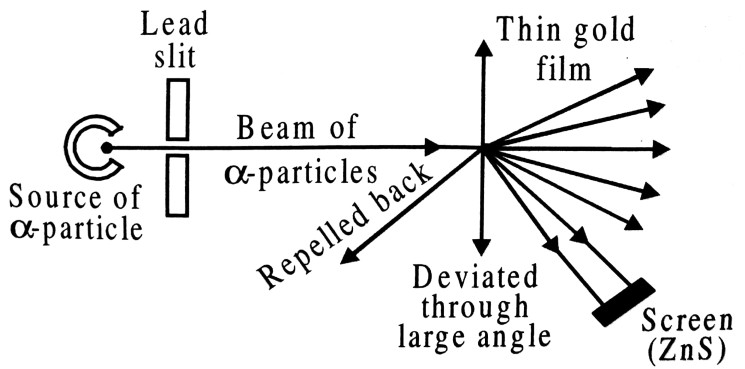Rutherford’s ∝-particle scattering experiment

The important inferences drawn from the experiment are :
(i) The most of the mass and the entire positive charge of the atom is concentrated in very small volume of the atom called nucleus.

Q.8. Sketch the energy level diagram for hydrogen atom. Mark the transition corresponding to Lyman and Balmer series.

Ans ⇒ (i) Lyman series : When an electron jumps from higher orbits to first orbit v when n1 = 1 and n2 = 2, 3, 4….. etc. then Lyman series is produced. The series is given by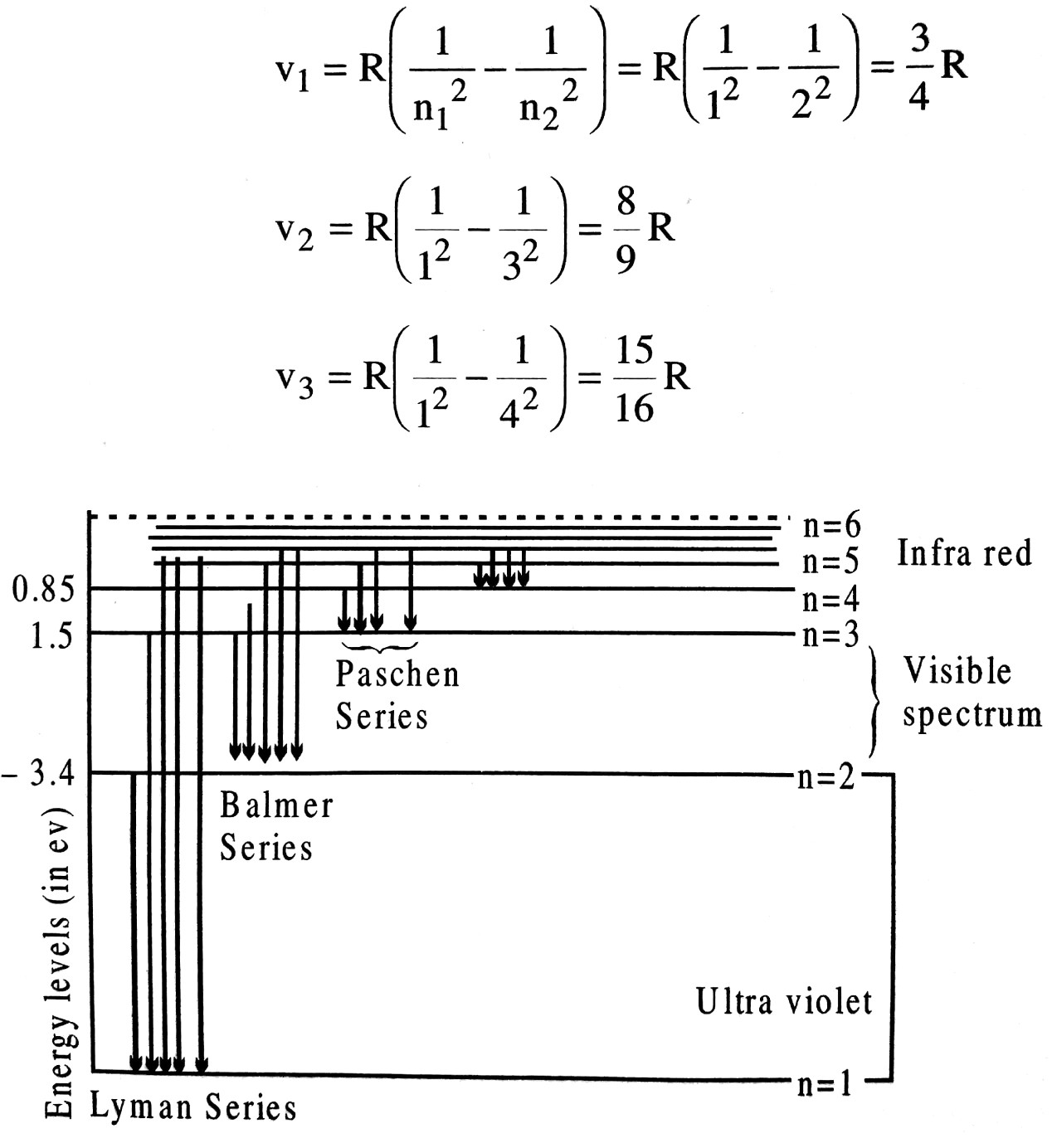There are called Lyman series and lie in the ultraviolet region.

(ii) Balmer series : When an electron jumps from an outer higher orbit to 2nd orbit v, n1 = 2 and n2 = 3,4,5…. etc. then Balmer series is produced.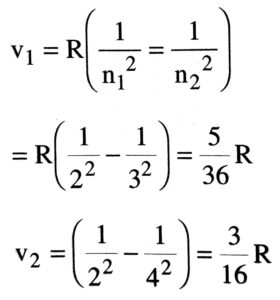Balmer series lies in the visible region.

Q.9. State Bohr’s postulates for atomic model.

Ans ⇒ (i) An atom consists of positively charged nucleus responsible for almost the entire mass of the atom.

(ii) The electrons revolve round the nucleus in certain permitted circular orbits of definite radii.

(iii) The permitted orbits are those in which the angular momentum of the electron is integral multiple of h/2π where h is Planck’s constant having value of 6.62 x 10-3 J sec. If m and and v are the mass and velocity of the electron in a permitted orbit of radius r, then
mvR = h/2π                  … (i)

where n is called principal quantum number and has the integral, values 1,2,3… The equation (i) is called Bohr’s quantisation condition.

(iv) When electrons revolve in permitted orbit, they do not radiate energy. Such orbits are called non-radiate or stationary orbits. Each stationary orbit is associated with a definite amount of energy.

(v) The energy is radiated when an electron jumps from lower to higher energy orbit.
If Ei and Ef are energies associated with the orbits of principle quantum number ni and nf respectively (ni > nf), the frequency of the radiation emitted is given by
hv = (Ei-Ef)                …(ii)

Q.10. State Bohr’s postulates of hydrogen atom. The wavelength of the first spectral line of Balmer series in hydrogen spectrum is 6563Å. Calculate the wavelength of the second line of the above series.

Ans ⇒ Bohr’s postulates :
(i) An electron in an atom revolves in a circular orbit around the nucleus. The force of attraction between the electron and the nucleus provides the necessary centripetal force for the circular motion.

(ii) The electron can revolve in those orbits for which its orbital angular momentum is an integral multiple of h/2π. The allowed orbits are given by
mvr = nh/2π                   …(i)
when ‘n’ is an integer and h is Planck’s constant. The integer n is known as the principal number and can take an integral value 1,2,3…. The numerical value of h is 6.624 x 10-34J.

(iii) The electron moving in allowed orbit does not radiate energy. Therefore, the total energy of the electron remains constant in an allowed orbit.

(iv) Electromagnetic radiation is emitted when the electron jumps from a higher orbit having energy En1 to a lower orbit having En2. The frequency v of the emitted radiation is given as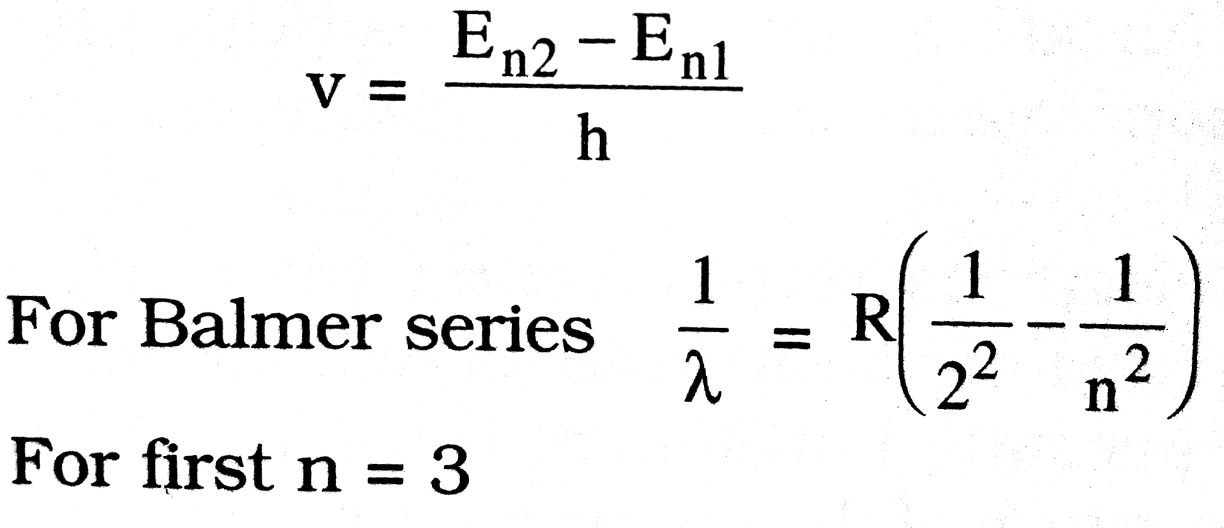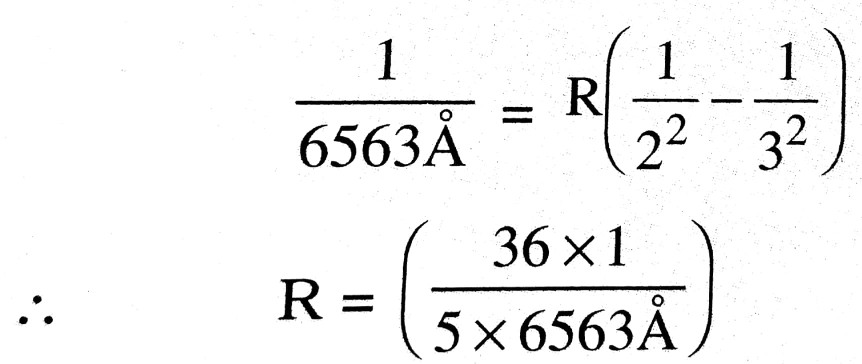For second line n = 4, therefore its wavelength λ is given as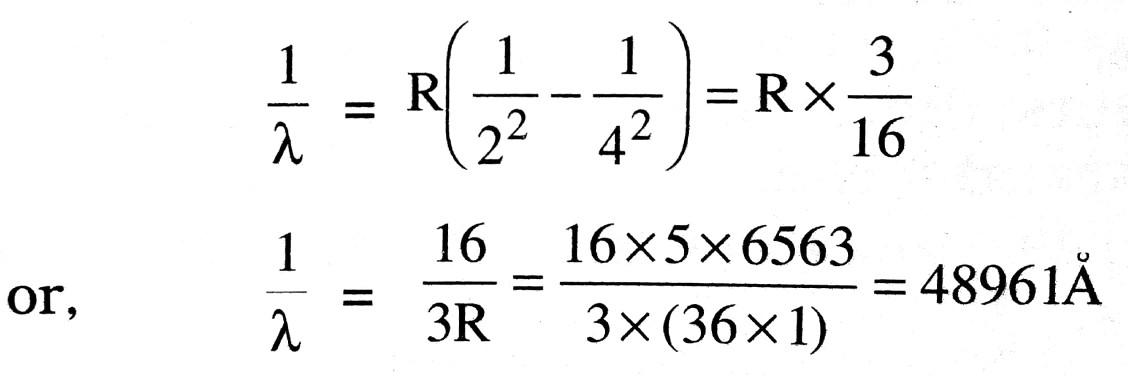Q.11. What are thermal neutrons ? Why are neutrons considered as ideal particles for nuclear fission ?

Ans ⇒ Such neutrons which have slowed down as a result of the collision against the hydrogen nuclei in a moderator are called thermal neutrons.
Neutrons are considered as ideal particle for nuclear fission because they are uncharged and therefore will not be repelled by any atomic nuclei at which they are thrown.

Q.12. Explain the term ‘distance and desert approach in Rutherford experiment and deduce the expression for it.

Ans ⇒ Distance of closest approach : In the experiment of Rutherford on the ∝-particles scattering the minimum distance between ∝-particle and the nucleus is knwn as distance of closet approach. It is represented by r0. At a certain distance r from the nucleus, K.E. of a-particle reduced to zero. The particle stops and it cannot go closer to the nucleus. It is repelled by the nucleus and therefore it retraces its path, turning through 180°. At the distance of closest approach the entire K.E. of a-particle is converted into electric potential energy.
Now, charge on r0 ∝-particle, q1=+2e
charge on nucleus, q2 = +Ze
Where z is the atomic number and +e charge on proton.
Electric potential at distance r0 due to the nucleus,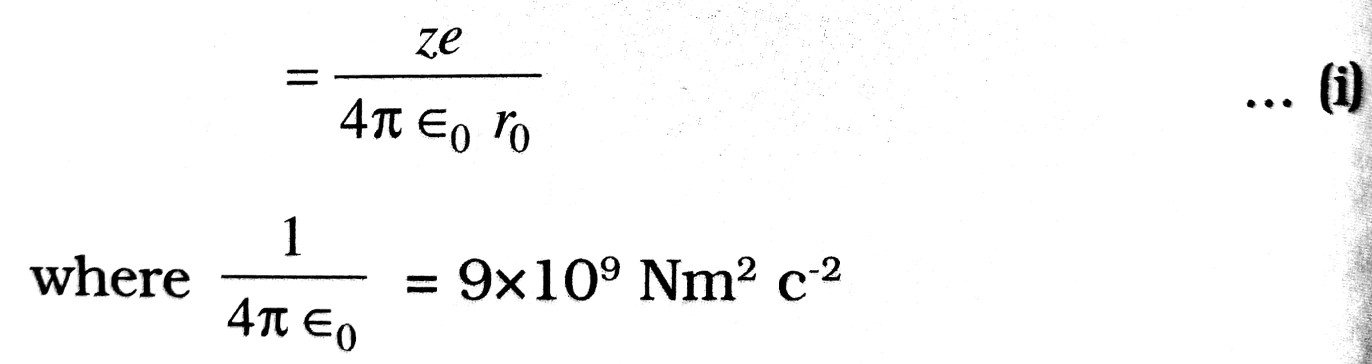∴    Potential energy of ∝-particle at this distance (r0) from the nucleus,
= Potential x Charge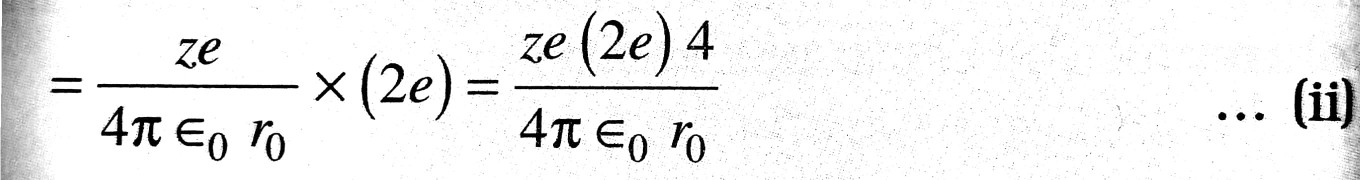Kinetic energy of ∝-particle of mass m moving with velocity v is given by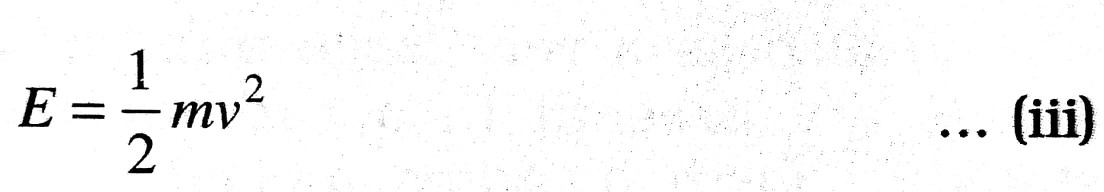If we neglect the loss of energy due to interaction of a-particle with the electrons then at the distance of closest approach, a K.E. ⇒ P.E.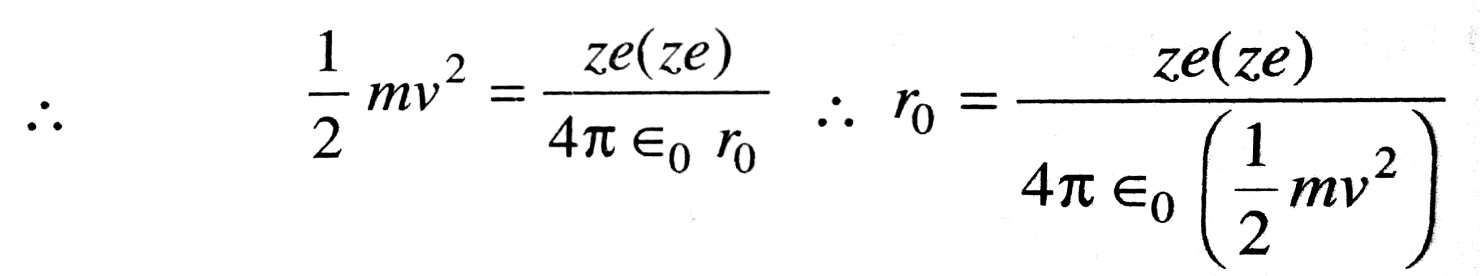Obviously, the radius of the nucleus must be smaller than the calculated value of r0 as an alpha particle cannot touch the periphery of the nuclenson Account of strong repulsion.

Q.13. Is Coulomb’s law true for atomic distances ?

Ans ⇒ Rutherford appreciated that when an ∝-particle passes through gold foil force acting between nucleus and ∝-particles is according to Coulomb’s law. It means the force of repulsion is inversely proportional to square of distance. Large the distance, smaller is the force so, ∝-particles passes from some region undeviated.
Gieger & Marsden came to the conclusion after experiment that force between nucleus and a particles is according to Coulomb’s law.

Q.14. What is atomic pile ?

Ans ⇒ It is an arragement is which large amount of energy released during fission is controlled. It is a type furnace in which uranium is used in form of a fuel. First atomic pile was made is 1942 is Chicago university lab in the supervision of Fermi.

## Class 12th physics Subjective question in English

 S.N Physics Short Type Question English Medium 1. ELECTRIC CHARGES AND FIELDS 2. LECTROSTATIC POTENTIAL AND CAPACITANCE 3. CURRENT ELECTRICITY 4. MOVING CHARGES AND MAGNETISM 5. MAGNETISM AND MATTER 6. ELECTROMAGNETIC INDUCTION 7. ALTERNATING CURRENT 8. ELECTROMAGNETIC WAVES 9. RAY OPTICS AND OPTICAL INSTRUMENTS 10. WAVE OPTICS 11. DUAL NATURE OF MATTER AND RADIATION 12. ATOMS 13. NUCLEI 14. SEMI CONDUCTOR ELECTRONICS 15. COMMUNICATION SYSTEMS